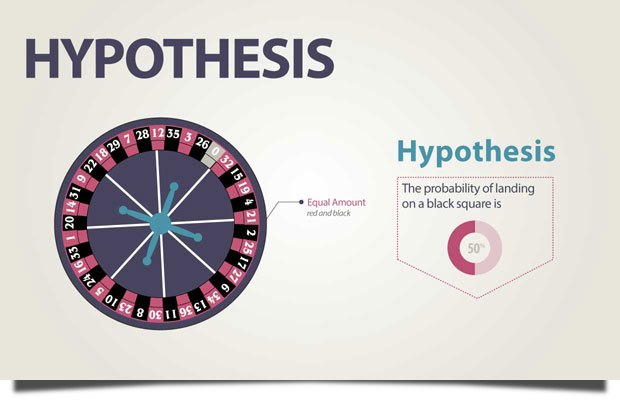# Hypothesis

A hypothesis is a statement about the value of a population parameter or a population model. You want to decide if the hypothesis is true or not.

- Triola (2009).

# ExampleThis example shows a roulette wheel and a statement/assumption that you have a 50% probability of landing on a black square. The statement/assumption is the hypothesis. This is just one example of a hypothesis.

## Related Terms

Null Hypothesis

Alternative Hypothesis